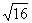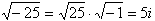Number of Solutions

The standard form of a quadratic equation is: ax 2 + bx + c = 0 . Solving quadratic equations will result in two, one or no real solutions. Since a quadratic equation contains a squared term, square roots will be needed to find solutions. Remember that the square root of a positive number produces a positive and negative answer. For example,= 4 and -4, since 42 = 16 and (-4) 2 = 16. This situation creates the two solution scenario. When taking the square root of zero, there is only one answer, 0. This situation creates the one solution scenario. When taking the square root of a negative number, an imaginary number is needed. For example,. This situation creates the no real solution scenario.

Listen